Select Page

### CAT 2022 Mensuration Practice Exercise-4

Welcome to this post on CAT 2022 Mensuration questions and answers. Understanding these questions is a bit of a task and needs lot of practice. In this area, you can find questions related to embedded areas, concatenated boundaries and concentric figures. Understanding of shapes and measures is a must if you want to attempt these. In fact, once you have a grasp over these different types of shapes, you will be able to comfortably solve questions from this area.

Directions for questions 1 & 2: Answer the questions based on the following information:

A cow is tethered at point A by a rope. Neither the rope nor the cow is allowed to enter the triangle ABC Angle BAC = 30· Also AB =AC =10m.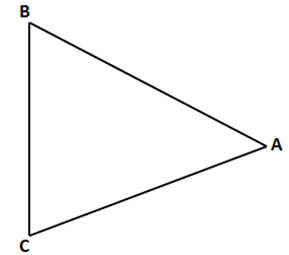Question 1: What is the area that can be grazed by the cow if the length of the rope is 8 m?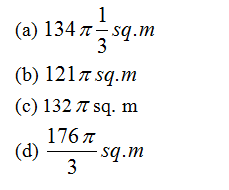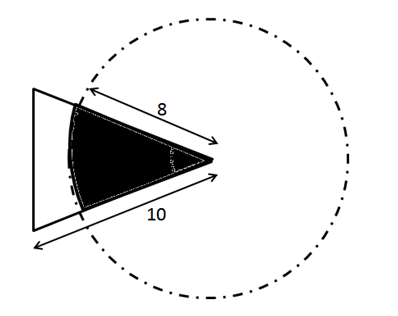The figure shows the prohibited area for the cow and the area the cow can graze. The length of the rope is 8 m whereas the length of the sides id 10 m. Hence the scenario is as explained in the figure above. The height of this triangle can be calculated and is approx 9.6m. So clearly greater than 8 m. So the area grazed by the cow = Area of the circle – Area of the sector of 30o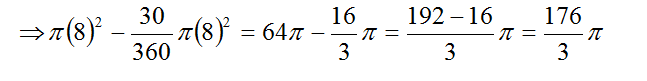Question 2: What is the area that can be grazed by the cow if the length of the rope is 12 m?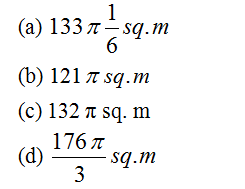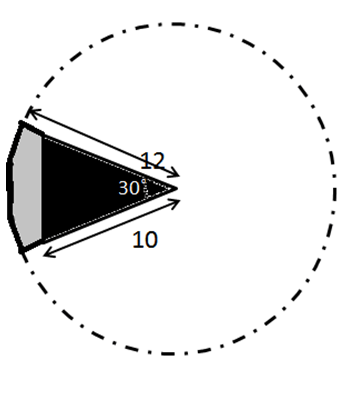Here the length of the rope is 12 m which is greater than the side of the triangle. Neither the cow nor the rope can enter the triangle. The area that can be grazed thereby is the area of the circle – the area of the sector of 30 degrees of the same circle which is equal to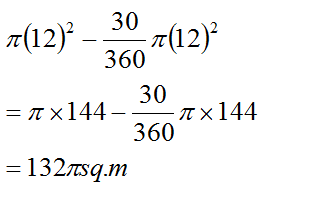Question 3: The figure below shows two concentric circles with centre O. PQRS is a square inscribed in the outer circle. It also circumscribes the inner circle, touching it at point B, C, D and A. What is the ratio of the perimeter of the outer circle to that of polygon ABCD?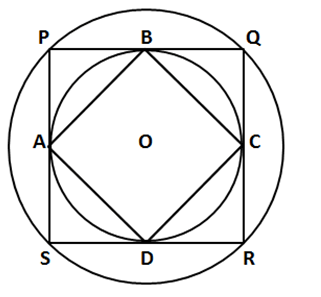(a) π/4
(b) 3π/2
(c) π/2
(d) π

Let r be the radius of the inner circle. A, B, C and D are points on its circumference where the sides of the square PQRS touch it imply that the sides are tangent to the circle. The radius and tangent are perpendicular at the point of contact. Thus if we join the radius OB and OC then OB is perpendicular to PQ, OC is perpendicular to QR, OB = OC = r, Hence OBQC is a square of side r. OQ is the diagonal = √2r = R = radius of outer circle.

BC is also the diagonal of OQ = R

Clearly ABCD, is a square of side R and radius of outer circle is also R

Perimeter of ABCD = 4R

Perimeter of circle = 2πR

Hence ratio = 2πR/4R = π/2

Question 4: What is the number of distinct triangles with integral valued sides and perimeter as 14?

(a) 6
(b) 5
(c) 4
(d) 3

An important property of the triangle is that the sum of its two sides should be greater than the third side

Let the sides of the triangle be a, b and c

Given a + b + c = 14 and by property of triangle:  a + b > c, b + c >a, c + a > b

Let one side be 1, sum of rest will be 13. Possible combinations are (1,12),(2,11),(3,10),(4,9),(5,8),(6,7)

In all the combinations the property of triangle is violated. Hence 1 cannot be the side of the triangle

Then if one side is 2, only combination satisfied is (6,6).

Accordingly, following 4 combinations can only be formed –

(4,4,6), (5,5,4), (6,5,3) and (6,6,2).

Question 5: A rectangular pool 20 meter wide and 60 meter long is surrounded by a walkway of uniform width. If the total area of the walkway is 516 square meter, how wide, in meter, is the walkway?

(a) 5
(b) 4·5
(c) 3
(d) 3·5

Let the width of the path be x meters.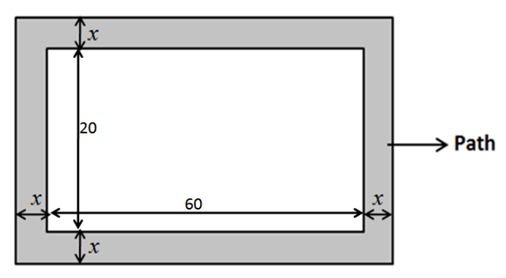Given, Area of the path = 516 m22

Area of the path = Area of the outer rectangle – Area of the pool

Area of pool = 60×20

Area of outer rectangle = (60 + 2x)(20+2x)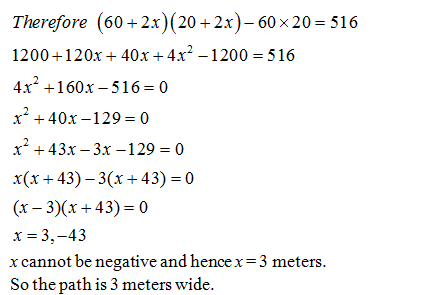Extra tips for CAT 2022 Mensuration Questions and Answers:

• In order to solve CAT Mensuration Questions and Answers successfully, nurush up the concepts of concentric circles and rectangles, concepts of circle such as: arcs, sectors, etc.
• Ideally, you should start the solution by first analyzing the question and dividing it into sub parts. Visualize how the various shapes are interconnected and which parameters are to be found.
• List the given parameters and unknown ones and map them to the respective shapes and formulas.
• Check the diagram drawn and whether it satisfies all the given conditions. Only then proceed further with the calculations. Remember! If you don’t get the diagram right, you will end up with the wrong answer.

Get Posts Like This Sent to your Email
Updates for Free Live sessions and offers are sent on mail. Don't worry: we do not send too many emails..:)
Get Posts Like This Sent to your Email
Updates for Free Live sessions and offers are sent on mail. Don't worry: we do not send too many emails..:)